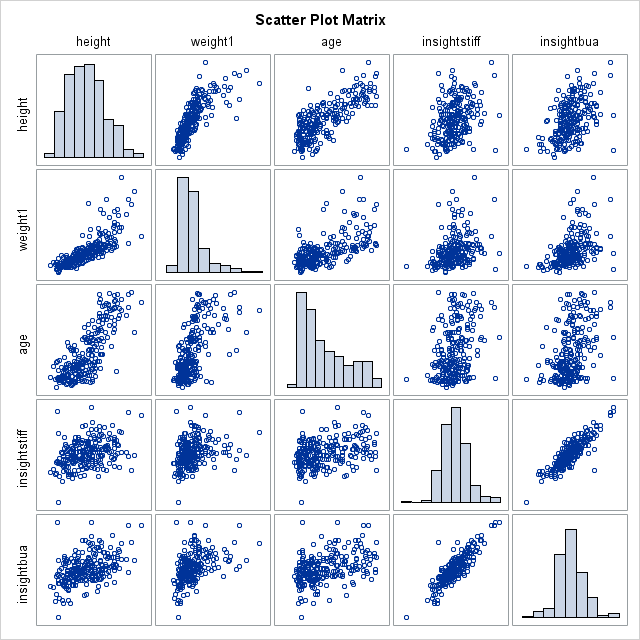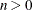## Correlation Matrix Plot Not Plotting All Variables

Hi,

I am trying to make correlation matrix plots using 6 variables: height, weight, age,  and the ultrasound measures stiffness index, bua, and sos. For some reason, I can't get the plot to include all the variables - it cuts off the last one, "Insightsos". Can you tell me what I can do to get SAS to plot all these variables in the matrix? I'm using SAS 9.4 M4 for Windows 7 64bit.

Thanks,

Cara

``````ods graphics on;
proc corr data=achilles_m nomiss plots=matrix(histogram) plots(maxpoints=none);
by winbonevisit;
where insight=1;
var Height Weight1 Age Insightstiff Insightbua Insightsos;
format winbonevisit winbonevisitf.;
run;
ods graphics off;``````1 ACCEPTED SOLUTION

Accepted Solutions

## Re: Correlation Matrix Plot Not Plotting All Variables

From the documentation:

NVAR=ALL | n

specifies the maximum number of variables in the VAR list to be displayed in the matrix plot, where. The NVAR=ALL option uses all variables in the VAR list. By default, NVAR=5.

3 REPLIES 3

## Re: Correlation Matrix Plot Not Plotting All Variables

From the documentation:

NVAR=ALL | n

specifies the maximum number of variables in the VAR list to be displayed in the matrix plot, where. The NVAR=ALL option uses all variables in the VAR list. By default, NVAR=5.

## Re: Correlation Matrix Plot Not Plotting All Variables

This worked, thank you!

## Re: Correlation Matrix Plot Not Plotting All Variables

Possible causes without seeing the data:

The variable is not numeric. check with Proc Contents or anything that displays column/ variable properties.

The variable is missing for all the records where insight=1 for one or more levels of the By variable.

One check would be Proc freq with insight*Insightsos on the tables statement with an identical By statement.

Insufficient space in the graphics area. You can test this by setting width and height options on the ODS Graphics to values such as

ods graphics on / width=10in height=10in;

to use a 10 inch by 10 inch display.

Do you get any tabular output for that variable?

Discussion stats
• 3 replies
• 4905 views
• 2 likes
• 3 in conversation